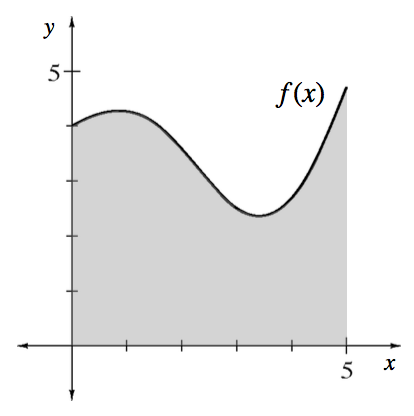### Home > APCALC > Chapter 8 > Lesson 8.3.1 > Problem8-102

8-102.

The graph at right shows $f(x)=\frac { 1 } { 2 }x \cos(x) + 4$ for $0 ≤ x ≤ 5$. Write an integral that will compute the volume of the solid when this region is rotated about:

1. The $x$-axis.

Vertical rectangles.

2. The $y$-axis.

Horizontal rectangles would be very difficult (because the inverse of $f$ is NOT a function). Use the Shell Method.

3. The line $x = 6$.

Recall that each cylindrical shell is a prism with dimensions: $\text{(base)(height)(width) }= (2πr)(f(x))(dx)$.
$(6 − x)$ represents the $r$ (radius) in the circumference part of the formula: $C = 2πr$. (Why?)

By shells: $V=2\pi \int_{0}^{5}(6-x)f(x)dx$

4. The line $y = -7$.

Vertical rectangles with a hole in the center.